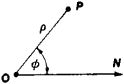# Polar Coordinates in the Plane

## Polar Coordinates in the Plane

two numbers that determine the position of a point relative to some fixed point O (the pole) and some fixed ray ON (the polar axis) issuing from the pole. The number ρ (radius vector) and the number ϕ (polar angle) are correspondingly equal to the distance from O to P and the angle between ON and OP (see Figure 1). The angle ϕ is sometimes called the amplitude, or phase, of the point P.Figure 1

In order to set up a one-to-one correspondence between points in the plane and pairs of polar coordinates, polar coordinates are usually restricted within the intervals 0 ≤ ρ < + ∞ and 0 ≤ ϕ < 2π; the polar angle of the pole is undefined. But if continuity is preferable to this single-valued property, that is, if it is desirable for the polar coordinates of a point to vary continuously as the point moves continuously, the quantity ϕ0 + kπ, where k is an arbitrary number and ϕ0 is the angle NOP, may be taken as the polar angle; the polar radius is then taken to be either positive or negative, depending on whether the direction of the ray OP coincides with or is opposite to the direction obtained as a result of rotating the ON axis through an angle equal to the selected value of the polar angle.

Site: Follow: Share:
Open / Close